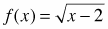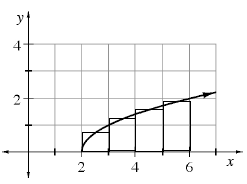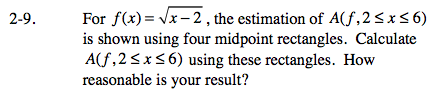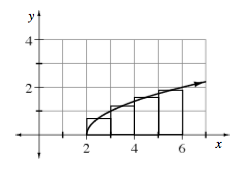### Home > CALC > Chapter 2 > Lesson 2.1.1 > Problem2-9

2-9.
1. For, the estimation of A(f, 2 ≤ x ≤ 6) is shown below using four midpoint rectangles. Calculate A(f, 2 ≤ x ≤ 6) using these rectangles. How reasonable is your result? Homework Help ✎A = (base)(height)
Base = 1 for all rectangles. Height is determined by the function, evaluated at midpoints.

$\text{The height of the smallest rectangle is }\sqrt{0.5}.$

$\text{The height of the largest rectangle is }\sqrt{3.5}.$